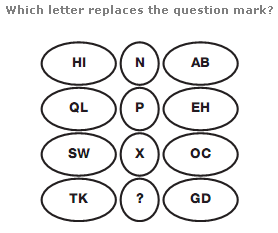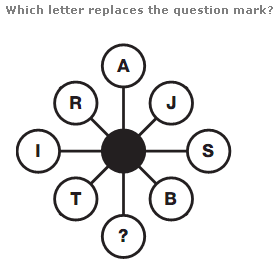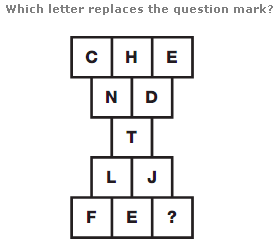# Puzzles - Missing letters puzzles

### Exercise :: Missing letters puzzlesAnswer : T Explanation : In each row, add together the numerical values of the pairs of letters to the left and right, then calculate their difference, putting the letter with this numerical value in the centre space.Answer : K Explanation : Start on the left hand side, and move clockwise around the diagram. Letters advance through the alphabet 9 letters at a time.Answer : M Explanation : Working in rows, from top to bottom, the sum of the numerical values of the letters follows the sequence 16, 18, 20, 22, 24.